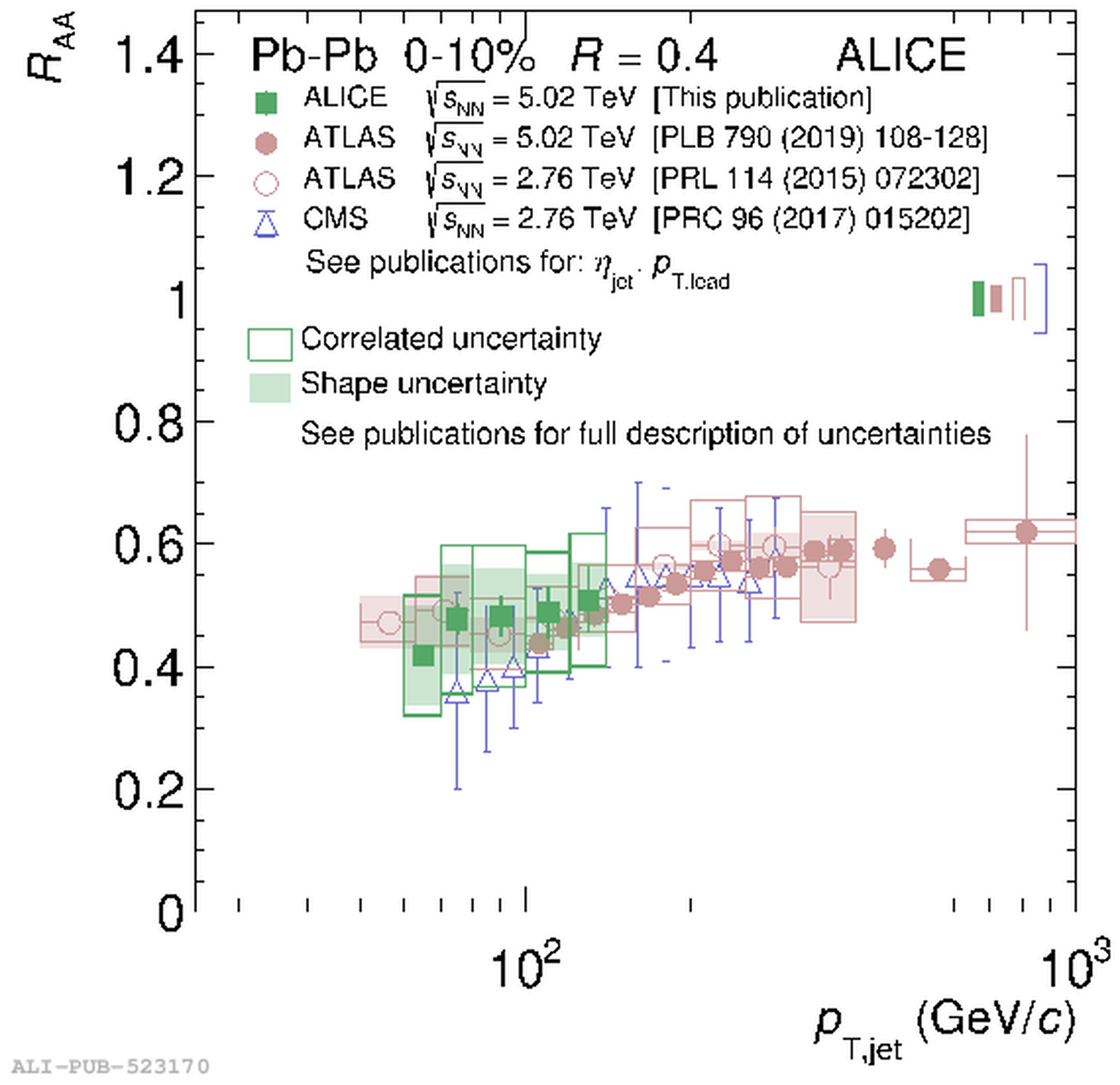# Figure 7

 Jet \Raa{} in 0-10\% central \PbPb{} collisions for $R=0.2$ (left) and $R=0.4$ (right) for all currently published experimental results. Closed markers denote $\sqrts=5.02$ TeV, and open markers denote $\sqrts=2.76$ TeV.